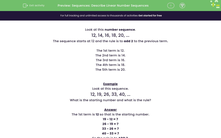# Sequences: Describe Linear Number Sequences

In this worksheet, students describe linear number sequences by giving the starting number and the rule.Key stage:  KS 2

Curriculum topic:   Algebra

Curriculum subtopic:   Know Linear Number Sequences

Popular topics:   Algebra worksheets, Ordering Numbers worksheets

Difficulty level:#### Worksheet Overview

Look at this number sequence.

12, 14, 16, 18, 20, ...

The sequence starts at 12 and the rule is to add 2 to the previous term.

The 1st term is 12.

The 2nd term is 14.

The 3rd term is 16.

The 4th term is 18.

The 5th term is 20.

Example

Look at this sequence.

12, 19, 26, 33, 40, ...

What is the starting number and what is the rule?

The 1st term is 12 so that is the starting number.

19 - 12 = 7

26 - 19 = 7

33 - 26 = 7

40 - 33 = 7

So the rule is to ADD 7.

### What is EdPlace?

We're your National Curriculum aligned online education content provider helping each child succeed in English, maths and science from year 1 to GCSE. With an EdPlace account you’ll be able to track and measure progress, helping each child achieve their best. We build confidence and attainment by personalising each child’s learning at a level that suits them.

Get started